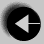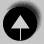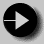## Weight Conversion with Command Line Argument

In this example, the number to be converted is supplied as part of the command to run the program. This can be a useful way of supplying a limited number of names or numbers to a program. We can run the program by typing program 1200 to convert 1200 lbs.

```
#include <stdio.h>

void print_converted(int pounds)
/* Convert U.S. Weight to Imperial and International
Units. Print the results */
{       int stones = pounds / 14;
int uklbs = pounds % 14;
float kilos_per_pound = 0.45359;
float kilos = pounds * kilos_per_pound;

printf("   %3d          %2d  %2d        %6.2f\n",
pounds, stones, uklbs, kilos);
}

main(int argc,char *argv[])
{       int pounds;

if(argc != 2)
{        printf("Usage: convert weight_in_pounds\n");
exit(1);
}

sscanf(argv, "%d", &pounds); /* Convert String to int */

printf(" US lbs      UK st. lbs       INT Kg\n");
print_converted(pounds);
}```

The main function definition has changed so that it takes two arguments, argc is a count of the number of arguments, and argv is an array of strings containing each of the arguments. The system creates these when the program is run.

Some other new concepts are introduced here.

if(argc != 2) Checks that the typed command has two elements, the command name and the weight in pounds.

exit(1); Leave the program. The argument 1 is a way of telling the operating system that an error has occurred. A 0 would be used for a successful exit.

sscanf(argv, "%d", &pounds)
Converts a string like "100" into an integer value stored in pounds. The argument is stored in argv as a string. sscanf works like scanf, but reads from a string instead of from the terminal.

The rest of the program is the same as the previous one.Go to Weight conversion withGo to IndexGo to Fibonacci series